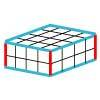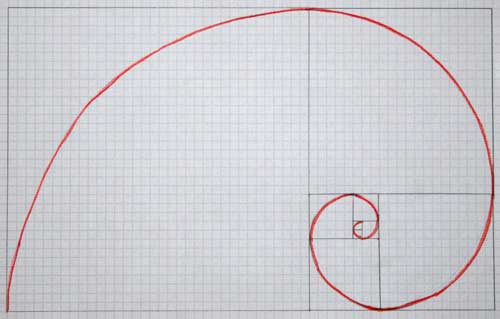#### You may also like### Cubes

How many faces can you see when you arrange these three cubes in different ways?### Bracelets

Investigate the different shaped bracelets you could make from 18 different spherical beads. How do they compare if you use 24 beads?### Making Cuboids

Let's say you can only use two different lengths - 2 units and 4 units. Using just these 2 lengths as the edges how many different cuboids can you make?

# Making Spirals

##### Age 7 to 11 Challenge Level:
There are lots of different spirals and lots of different ways of creating them.
Here are two different ways.
Try them out and see whether you can create some more of your own.
Here is a link to another NRICH activity with instructions to create Archimedes' Spiral.

And now for another one: a Golden or Fibonacci Spiral.Here are the instructions:
Take a piece of A$4$ squared or graph paper. The best one to use is one that is divided into 5mm squares. Put the paper on your desk so that its longest side is horizontal.
Start by drawing a square with a side of one about $10$ squares up from the bottom edge of the paper and $15$ squares in from the right hand side.
Draw another square with a side of one above it.
Now draw a square of side two to the right of your first two squares and then a square of side $3$ above that.
You can now start to draw your spiral.
Each square has a quarter of a circle in it which joins one corner of the square to its opposite corner.
Can you see where to draw your next square and curve?
The sizes of your squares follow the Fibonacci sequence: $1$, $1$,$2$, $3$, $5$, $8$, $13$, $21$, $34$. Can you see why? You will run out of space on your paper when you get to $21$ or $34$ - it just depends on exactly where you positioned your first square on the paper.

What do you notice about these spirals? Are they similar?

These two spirals are made by starting in the centre and building outwards.

Can you create any more rules for making your own spirals?